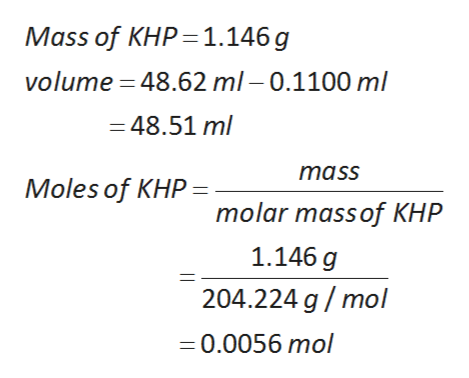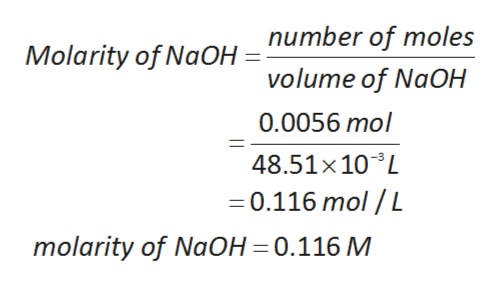# A solution of sodium hydroxide is standardized against potassium hydrogen phthalate (molecular mass = 204.224 g mol-1). From the following data, calculate the molarity of the NaOH solution. The mass of the KHP is 1.146 g. Before the titration the buret reading was 0.1100 mL and afterwards it was 48.62 mL. A 77.90 mL sample of a solution of sulfuric acid, H2SO4, is neutralized by 119.03 mL of the NaOH solution from the problem above. Calculate the molarity of the sulfuric acid solution.

Question
5 views

A solution of sodium hydroxide is standardized against potassium hydrogen phthalate (molecular mass = 204.224 g mol-1). From the following data, calculate the molarity of the NaOH solution. The mass of the KHP is 1.146 g. Before the titration the buret reading was 0.1100 mL and afterwards it was 48.62 mL.

A 77.90 mL sample of a solution of sulfuric acid, H2SO4, is neutralized by 119.03 mL of the NaOH solution from the problem above. Calculate the molarity of the sulfuric acid solution.

check_circle

Step 1

Chemical reaction between sodium hydroxide and KHP as follows:

Step 2

the value of  mass KHP is 1.146 g  and  the volume  is 48.51 ml. The moles of KHP is 0.0056 mol.help_outlineImage TranscriptioncloseMass of KHP 1.146 g volume 48.62 ml- 0.1100 ml 48.51 ml mass Moles of KHP - molar mass of KHP 1.146 g 204.224 g/mol 0.0056 mol fullscreen
Step 3

1 mole of KHP react with 1 mole of NaOH

0.0056 moles of KHP react wit...help_outlineImage Transcriptionclosenumber of moles Molarity of NaOH volume of NaOH 0.0056 mol 48.51x10L 0.116 mol / L molarity of NaOH =0.116 M fullscreen

### Want to see the full answer?

See Solution

#### Want to see this answer and more?

Solutions are written by subject experts who are available 24/7. Questions are typically answered within 1 hour.*

See Solution
*Response times may vary by subject and question.
Tagged in

### Chemistry